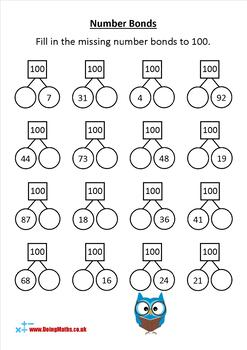Number Bonds Worksheet Set - Arithmetic PracticeK - 3rd
Subjects
Standards
Resource Type
Formats Included
• Zip
Pages
10 pages

Description

Get your students practising their number bonds with these ten worksheets featuring number bonds to ten, twenty, one hundred, one thousand and decimal number bonds to one. Designed to cover all levels of ability within your classroom, from those starting out, through to higher ability who need stretching with higher numbers and/or decimals.

The set contains:

• Two worksheets featuring basic integer number bonds to ten
• Two worksheets featuring basic integer number bonds to twenty
• Three worksheets featuring number bonds to one hundred, differentiated from multiples of ten, through to multiples of five and finally any integer pair
• One worksheet featuring number bonds to one thousand
• Two bonus worksheets featuring decimal number bonds to one, differentiated from one decimal place through to two decimal places.

All files are in PDF format with answer sheets included.

More DoingMaths activities and info

Related Resources

Cafe Menu Maths - Arithmetic and money practice: UK Version or US Version

Arithmetic Riddles Bundle - Fun arithmetic practise

This purchase includes a license for one teacher for use within their classroom. Copying for use by more than one teacher or within a department is prohibited without purchasing the correct number of licenses.

This resource may not be uploaded to the internet in any form with the exception of a secure, password-protected system for student use.

Total Pages
10 pages
Included
Teaching Duration
N/A
Report this Resource to TpT
Reported resources will be reviewed by our team. Report this resource to let us know if this resource violates TpT’s content guidelines.

Standards

to see state-specific standards (only available in the US).
Fluently add and subtract within 20 using mental strategies. By end of Grade 2, know from memory all sums of two one-digit numbers.
Add and subtract within 20, demonstrating fluency for addition and subtraction within 10. Use strategies such as counting on; making ten (e.g., 8 + 6 = 8 + 2 + 4 = 10 + 4 = 14); decomposing a number leading to a ten (e.g., 13 - 4 = 13 - 3 - 1 = 10 - 1 = 9); using the relationship between addition and subtraction (e.g., knowing that 8 + 4 = 12, one knows 12 - 8 = 4); and creating equivalent but easier or known sums (e.g., adding 6 + 7 by creating the known equivalent 6 + 6 + 1 = 12 + 1 = 13).
For any number from 1 to 9, find the number that makes 10 when added to the given number, e.g., by using objects or drawings, and record the answer with a drawing or equation.
Solve addition and subtraction word problems, and add and subtract within 10, e.g., by using objects or drawings to represent the problem.